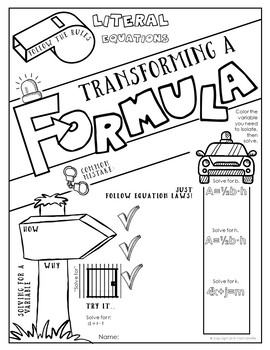# Formulas Doodle NotesSubject
Resource Type
File Type
PDF (2 MB|2 plus answer keys & info)
Standards
\$3.25
• Product Description
• Standards

Using a formula, transforming a formula (literal equations): 2 page visual interactive "doodle notes" set -

When students color or doodle in math class, it activates both hemispheres of the brain at the same time. There are proven benefits of this cross-lateral brain activity:

- new learning

- relaxation (less math anxiety)

- visual connections

- better memory & retention of the content!

Students fill in the sheets, answer the questions, and color, doodle or embellish. Then, they can use it as a study guide later on. Graphic doodle and sketch notes take full advantage of Dual Coding Theory (the way brains process visual and linguistic information) to maximize retention.

Content includes:

- What are formulas (and some samples)

- How to use them

- Literal Equations (transforming a formula)

- How to solve for a variable / isolate a variable

- Visual memory triggers and graphics & tasks to boost retention

- practice & examples

Visual note taking strategies like sketch notes or doodle notes are based on dual coding theory.  When we can blend the text input with graphic/visual input, the student brain processes the information differently and can more easily convert the new learning into long-term memory.

This strategy also integrates the left and right hemispheres of the brain to increase focus, learning, and retention!

Loving the doodle notes? Grab the full book of Pre-Algebra doodle notes here:

Pre Algebra Doodle Note Book

Solve linear equations in one variable.
Use variables to represent quantities in a real-world or mathematical problem, and construct simple equations and inequalities to solve problems by reasoning about the quantities.
Evaluate expressions at specific values of their variables. Include expressions that arise from formulas used in real-world problems. Perform arithmetic operations, including those involving whole-number exponents, in the conventional order when there are no parentheses to specify a particular order (Order of Operations). For example, use the formulas V = s³ and A = 6 s² to find the volume and surface area of a cube with sides of length s = 1/2.
Total Pages
2 plus answer keys & info